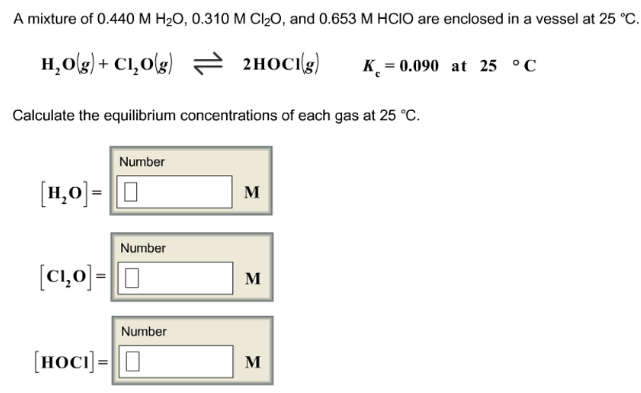# Problem: A mixture of 0.440 M H 2O, 0.310 M Cl2O, and 0.653 M HCIO are enclosed in a vessel at 25°C. H2O(g) + Cl2O(g) ⇌ 2HOCl(g)            K c = 0.090 at 25°C Calculate the equilibrium concentrations of each gas at 25°C.

🤓 Based on our data, we think this question is relevant for Professor Love's class at SDSU.

###### FREE Expert Solution###### Problem Details

A mixture of 0.440 M H 2O, 0.310 M Cl2O, and 0.653 M HCIO are enclosed in a vessel at 25°C.

H2O(g) + Cl2O(g) ⇌ 2HOCl(g)            K c = 0.090 at 25°C

Calculate the equilibrium concentrations of each gas at 25°C.